## 5.356. soft_all_equal_max_var

Origin
Constraint

$\mathrm{𝚜𝚘𝚏𝚝}_\mathrm{𝚊𝚕𝚕}_\mathrm{𝚎𝚚𝚞𝚊𝚕}_\mathrm{𝚖𝚊𝚡}_\mathrm{𝚟𝚊𝚛}\left(𝙽,\mathrm{𝚅𝙰𝚁𝙸𝙰𝙱𝙻𝙴𝚂}\right)$

Arguments
 $𝙽$ $\mathrm{𝚍𝚟𝚊𝚛}$ $\mathrm{𝚅𝙰𝚁𝙸𝙰𝙱𝙻𝙴𝚂}$ $\mathrm{𝚌𝚘𝚕𝚕𝚎𝚌𝚝𝚒𝚘𝚗}\left(\mathrm{𝚟𝚊𝚛}-\mathrm{𝚍𝚟𝚊𝚛}\right)$
Restrictions
 $𝙽\ge 0$ $𝙽\le |\mathrm{𝚅𝙰𝚁𝙸𝙰𝙱𝙻𝙴𝚂}|$ $\mathrm{𝚛𝚎𝚚𝚞𝚒𝚛𝚎𝚍}$$\left(\mathrm{𝚅𝙰𝚁𝙸𝙰𝙱𝙻𝙴𝚂},\mathrm{𝚟𝚊𝚛}\right)$
Purpose

Let $M$ be the number of occurrences of the most often assigned value to the variables of the $\mathrm{𝚅𝙰𝚁𝙸𝙰𝙱𝙻𝙴𝚂}$ collection. $𝙽$ is less than or equal to the total number of variables of the $\mathrm{𝚅𝙰𝚁𝙸𝙰𝙱𝙻𝙴𝚂}$ collection minus $M$ (i.e., $𝙽$ is less than or equal to the minimum number of variables that need to be reassigned in order to obtain a solution where all variables are assigned a same value).

Example
$\left(1,〈5,1,5,5〉\right)$

Within the collection $〈5,1,5,5〉$, 3 is the number of occurrences of the most assigned value. Consequently, the $\mathrm{𝚜𝚘𝚏𝚝}_\mathrm{𝚊𝚕𝚕}_\mathrm{𝚎𝚚𝚞𝚊𝚕}_\mathrm{𝚖𝚊𝚡}_\mathrm{𝚟𝚊𝚛}$ constraint holds since the argument $𝙽=1$ is less than or equal to the total number of variables 4 minus 3.

Typical
 $𝙽>0$ $𝙽<|\mathrm{𝚅𝙰𝚁𝙸𝙰𝙱𝙻𝙴𝚂}|$ $𝙽<|\mathrm{𝚅𝙰𝚁𝙸𝙰𝙱𝙻𝙴𝚂}|/10+2$ $|\mathrm{𝚅𝙰𝚁𝙸𝙰𝙱𝙻𝙴𝚂}|>1$
Symmetries
• $𝙽$ can be decreased to any value $\ge 0$.

• Items of $\mathrm{𝚅𝙰𝚁𝙸𝙰𝙱𝙻𝙴𝚂}$ are permutable.

• All occurrences of two distinct values of $\mathrm{𝚅𝙰𝚁𝙸𝙰𝙱𝙻𝙴𝚂}.\mathrm{𝚟𝚊𝚛}$ can be swapped; all occurrences of a value of $\mathrm{𝚅𝙰𝚁𝙸𝙰𝙱𝙻𝙴𝚂}.\mathrm{𝚟𝚊𝚛}$ can be renamed to any unused value.

Algorithm
Counting
 Length ($n$) 2 3 4 5 6 7 8 Solutions 15 148 1905 30006 555121 11758048 280310337

Number of solutions for $\mathrm{𝚜𝚘𝚏𝚝}_\mathrm{𝚊𝚕𝚕}_\mathrm{𝚎𝚚𝚞𝚊𝚕}_\mathrm{𝚖𝚊𝚡}_\mathrm{𝚟𝚊𝚛}$: domains $0..n$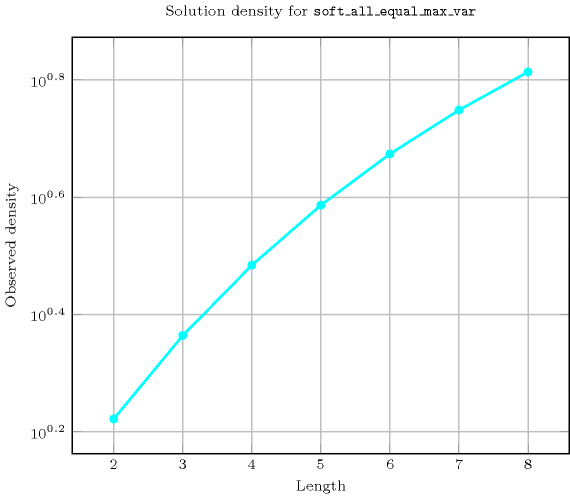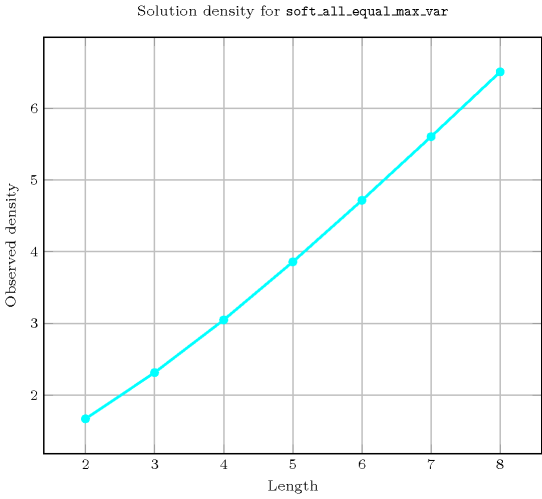Length ($n$)2345678
Total1514819053000655512111758048280310337
 Parameter value

09646257776117649209715243046721
16606207770117642209714443046712
2-245407620117390209675243046136
3--1206120113610208852043030008
4---72083790199248042771960
5----5040134568040194000
6-----4032024811920
7------362880

Solution count for $\mathrm{𝚜𝚘𝚏𝚝}_\mathrm{𝚊𝚕𝚕}_\mathrm{𝚎𝚚𝚞𝚊𝚕}_\mathrm{𝚖𝚊𝚡}_\mathrm{𝚟𝚊𝚛}$: domains $0..n$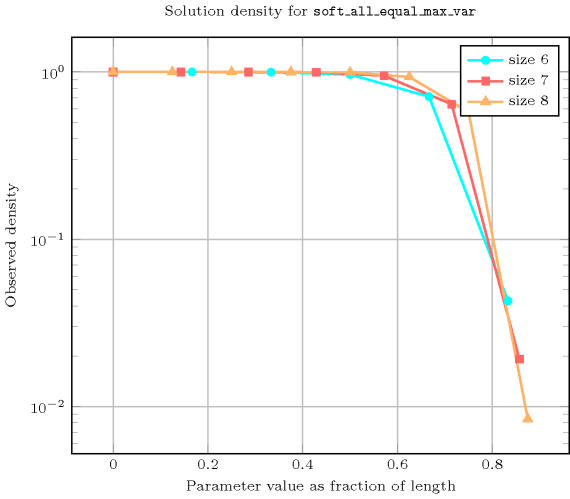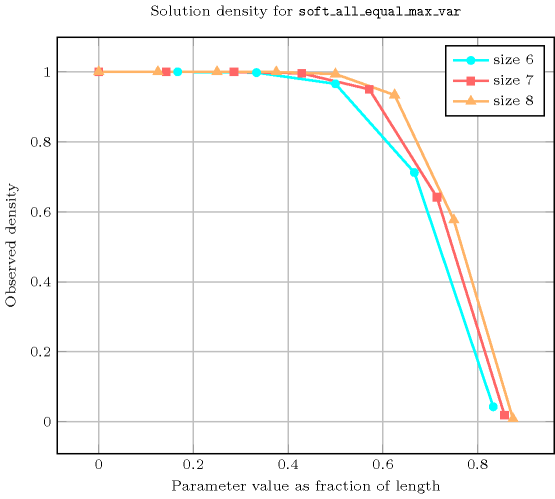Keywords
Arc input(s)

$\mathrm{𝚅𝙰𝚁𝙸𝙰𝙱𝙻𝙴𝚂}$

Arc generator
$\mathrm{𝐶𝐿𝐼𝑄𝑈𝐸}$$↦\mathrm{𝚌𝚘𝚕𝚕𝚎𝚌𝚝𝚒𝚘𝚗}\left(\mathrm{𝚟𝚊𝚛𝚒𝚊𝚋𝚕𝚎𝚜}\mathtt{1},\mathrm{𝚟𝚊𝚛𝚒𝚊𝚋𝚕𝚎𝚜}\mathtt{2}\right)$

Arc arity
Arc constraint(s)
$\mathrm{𝚟𝚊𝚛𝚒𝚊𝚋𝚕𝚎𝚜}\mathtt{1}.\mathrm{𝚟𝚊𝚛}=\mathrm{𝚟𝚊𝚛𝚒𝚊𝚋𝚕𝚎𝚜}\mathtt{2}.\mathrm{𝚟𝚊𝚛}$
Graph property(ies)
$\mathrm{𝐌𝐀𝐗}_\mathrm{𝐍𝐒𝐂𝐂}$$\le |\mathrm{𝚅𝙰𝚁𝙸𝙰𝙱𝙻𝙴𝚂}|-𝙽$

Graph model

We generate an initial graph with binary equalities constraints between each vertex and its successors. The graph property states that $𝙽$ is less than or equal to the difference between the total number of vertices of the initial graph and the number of vertices of the largest strongly connected component of the final graph.

Parts (A) and (B) of Figure 5.356.1 respectively show the initial and final graph associated with the Example slot. Since we use the $\mathrm{𝐌𝐀𝐗}_\mathrm{𝐍𝐒𝐂𝐂}$ graph property we show one of the largest strongly connected components of the final graph.

##### Figure 5.356.1. Initial and final graph of the $\mathrm{𝚜𝚘𝚏𝚝}_\mathrm{𝚊𝚕𝚕}_\mathrm{𝚎𝚚𝚞𝚊𝚕}_\mathrm{𝚖𝚊𝚡}_\mathrm{𝚟𝚊𝚛}$ constraint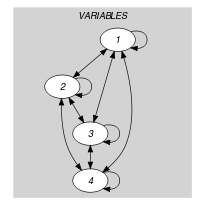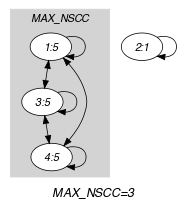(a) (b)